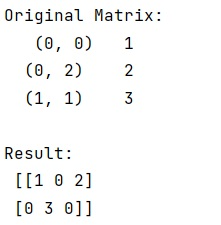# How to generate a dense matrix from a sparse matrix in NumPy?

Learn, how to generate a dense matrix from a sparse matrix in NumPy?
Submitted by Pranit Sharma, on February 17, 2023

NumPy is an abbreviated form of Numerical Python. It is used for different types of scientific operations in python. Numpy is a vast library in python which is used for almost every kind of scientific or mathematical operation. It is itself an array which is a collection of various methods and functions for processing the arrays.

## Generating a dense matrix from a sparse matrix in NumPy

Suppose that we are given a sparse matrix and we need to create a dense matrix from this sparse matrix using numpy.

For this purpose, we will use todense() on our sparse matrix which will directly convert it into a dense matrix.

This method returns a dense matrix representation of this matrix.

A NumPy matrix object with the same shape and containing the same data represented by the sparse matrix, with the requested memory order. If out (parameter) was passed and was an array (rather than a numpy.matrix()), it will be filled with the appropriate values and returned wrapped in a numpy.matrix() object that shares the same memory.

Let us understand with the help of an example,

## Python code to generate a dense matrix from a sparse matrix in NumPy

```# Import numpy
import numpy as np

from scipy.sparse import csr_matrix

# Creating a sparse matrix
A = csr_matrix([[1,0,2],[0,3,0]])

# Display original matrix
print("Original Matrix:\n",A,"\n")

# Converting sparse matrix to dense matrix
res = A.todense()

# Display result
print("Result:\n",res)
```

Output: